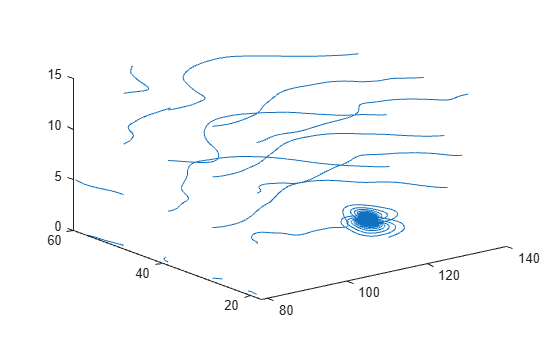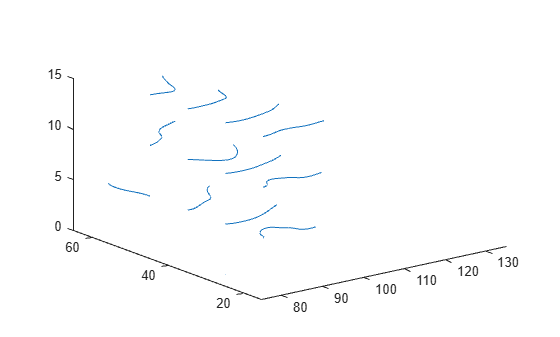# stream3

Compute 3-D streamline data

## Syntax

``XYZ = stream3(X,Y,Z,U,V,W,startX,startY,startZ)``
``XYZ = stream3(U,V,W,startX,startY,startZ)``
``XYZ = stream3(___,options)``

## Description

example

````XYZ = stream3(X,Y,Z,U,V,W,startX,startY,startZ)` returns streamline data as a 2-D matrix of vector fields. The inputs `X`, `Y`, and `Z` are vector data coordinates, `U`, `V`, and `W` are vector data, and `startX`, `startY`, and `startZ` are the starting positions of the streamlines.```
````XYZ = stream3(U,V,W,startX,startY,startZ)` uses the default coordinate data for `U`, `V`, and `W`. The (x,y,z) location for each element in `U`, `V`, and `W` is based on the column, row, and page index, respectively.```

example

````XYZ = stream3(___,options)` computes 3-D streamline data using the specified options, defined as a one- or two-element vector with the form `step` or `[step maxvert]`, where `step` is the step size for interpolating the vector data and `maxvert` is the maximum number of vertices in a streamline. Use this argument with any of the input argument combinations from the previous syntaxes.```

## Examples

collapse all

Load the `wind` data set which contains measurements of air current over regions of North America.

• 3-D arrays `x`, `y`, and `z` represent the locations of air current measurements.

• 3-D arrays `u`, `v`, and `w` represent the velocity of the air current in 3-D vector fields.

Define the starting position of 16 hypothetical particles. In this case, the particles all start at `x` = 80 and have starting `y` positions ranging from 20 to 50 and starting `z` positions ranging from 0 to 15.

```load wind [startX,startY,startZ] = meshgrid(80,20:10:50,0:5:15);```

Compute the 3-D streamline vertex data for a hypothetical particle placed into the air current at the collection of starting positions in `startX`, `startY`, and `startZ`.

`verts = stream3(x,y,z,u,v,w,startX,startY,startZ);`

Visualize the 3-D volume of vector fields with `streamline`. Return the line objects in the variable `lineobj`, so you can change their properties later.

```lineobj = streamline(verts); view(3)```To change aspects of a particular line, set properties on one of the returned line objects. For example, change the color of the tenth line to green and change its thickness to `3`.

```lineobj(10).Color = "g"; lineobj(10).LineWidth = 3;```Load the `wind` data set, which contains measurements of air current over regions of North America.

• 3-D arrays `x`, `y`, and `z` represent the locations of air current measurements.

• 3-D arrays `u`, `v`, and `w` represent the velocity of the air current in 3-D vector fields.

Define the starting position of 16 hypothetical particles. In this case, the particles all start at `x` = 80 and have starting `y` positions ranging from 20 to 50 and starting `z` positions ranging from 0 to 15.

```load wind [startX,startY,startZ] = meshgrid(80,20:10:50,0:5:15);```

Decrease the streamline resolution by increasing the step size from the default of 0.1 to 3.

`step = 3;`

Compute the 3-D streamline vertex data for a hypothetical particle placed into the air current at the collection of starting positions in `startX`, `startY`, and `startZ`.

`verts = stream3(x,y,z,u,v,w,startX,startY,startZ,step);`

Visualize the 3-D volume of vector fields with `streamline`. The larger step size results in a lower resolution streamline.

```streamline(verts) view(3)```Load the `wind` data set, which contains measurements of air current over regions of North America.

• 3-D arrays `x`, `y`, and `z` represent the locations of air current measurements.

• 3-D arrays `u`, `v`, and `w` represent the velocity of the air current in 3-D vector fields.

Define the starting position of 16 hypothetical particles. In this case, the particles all start at `x` = 80 and have starting `y` positions ranging from 20 to 50 and starting `z` positions ranging from 0 to 15.

```load wind [startX,startY,startZ] = meshgrid(80,20:10:50,0:5:15);```

Increase the streamline resolution by decreasing the step size from the default of 0.1 to 0.01.

`step = 0.01;`

Set the maximum number of vertices so that computation ends after the first 1,000 vertices are calculated.

`maxvert = 1000;`

Compute the 3-D streamline vertex data for a hypothetical particle placed into the air current at the collection of starting positions in `startX`, `startY`, and `startZ`.

`verts = stream3(x,y,z,u,v,w,startX,startY,startZ,[step maxvert]);`

Visualize the 3-D volume of vector fields with `streamline`. Show the full range of data values by setting the axis limits. The streamlines end after 1,000 vertices are calculated, so the streamlines stop before showing the full range of data.

```streamline(verts) xlim([75 135]) ylim([15 65]) zlim([0 15]) view(3)```## Input Arguments

collapse all

x-axis coordinates of vector data, specified as a 3-D array. It must be monotonic, but does not need to be uniformly spaced. `X` must be the same size as `Y`, `Z`, `U`, `V`, and `W`.

You can use the `meshgrid` function to create `X`.

y-axis coordinates of vector data, specified as a 3-D array. It must be monotonic, but does not need to be uniformly spaced. `Y` must be the same size as `X`, `Z`, `U`, `V`, and `W`.

You can use the `meshgrid` function to create `Y`.

z-axis coordinates of vector data, specified as a 3-D array. It must be monotonic, but does not need to be uniformly spaced. `Z` must be the same size as `X`, `Y`, `U`, `V`, and `W`.

You can use the `meshgrid` function to create `Z`.

x-components of vector data, specified as a 3-D array. `U` must be the same size as `X`, `Y`, `Z`, `V`, and `W`.

y-components of vector data, specified as a 3-D array. `V` must be the same size as `X`, `Y`, `Z`, `U`, and `W`.

z-components of vector data, specified as a 3-D array. `W` must be the same size as `X`, `Y`, `Z`, `U`, and `V`.

x-axis streamline starting positions, specified as a vector or matrix. `startX` must be a scalar or be the same size as `startY` and `startZ`.

y-axis streamline starting positions, specified as a vector or matrix. `startY` must be a scalar or be the same size as `startX` and `startZ`.

z-axis streamline starting positions, specified as a vector or matrix. `startZ` must be a scalar or be the same size as `startX` and `startY`.

Streamline options, specified as a one- or two-element vector with one of the following forms:

• `step`

• `[step maxvert]`

`step` is the step size used to adjust the streamline resolution and determine the vertex locations for which streamline velocity is interpolated. `maxvert` is the maximum number of vertices calculated for a streamline before computation is complete.

The default step-size is `0.1`, and the default maximum number of vertices in a streamline is `10,000`.

## Version History

Introduced before R2006a